Force Influence By Mass

Particle Flow allows the user to control the influence of certain Force-related Operators via MAXScript-generated particle channels. This is called Particle Wiring and is a rather hidden option in the Particle Flow User Interface.
The following tutorial shows how to calculate a mass value for each particle and control the influence of a Wind force to get a more realistic behavior where light particles are blown away while heavy ones stay almost unaffected.

 Natural Language - Setup
• Generate Particles on a surface without any speed or rotation, just position.

• Add a Scale Operator to vary the size of the particles.

• Add a Script Operator which will read the size of the particles generated by the Scale Operator.

• Add a Force Operator

• Create A Wind Space Warp, add to the Force Operator

• Enable Script Wiring (see below)

• Edit the script of the Script Operator to calculate the influence value for the Force Operator:

 Natural Language - Script

• Enable the Scale and Force channels

• For each particle, get the Scale value (the size of the particle)

• Calculate the influence value

• Assign to the Force channel.

 Particle View SetupThe Particle View setup features a Scale operator which defines variable sizes for the particles.

In order to get the Script Wiring, you have to do the following:

A: Add a Script Operator BEFORE the Force Operator to generate the Particle Float channel values needed to control the Force Influence.

B: Right-click the Force Operator and check the "Use Script Wiring" option.

C: This enables a new additional rollout in the Force Operator which lets you enable the Influence Control through the Float channel.

 Complete Script

on ChannelsUsed pCont do
(
pCont.useScale = true
pCont.useFloat = true
)

on Init pCont do
(
)

on Proceed pCont do
(
count = pCont.NumParticles()
for i in 1 to count do
(
pCont.particleIndex = i
pCont.particleFloat = 1.0/(pCont.particleScale^3)
)
)

on Release pCont do
(
)

 Final Result

The Wind Force affects the particles depending on their size which is proportional to their mass assuming that the density is constant.
Small (and thus lightweight) particles are affected more than heavy ones.

Click here for a preview AVI (1MB, DivX 5.2)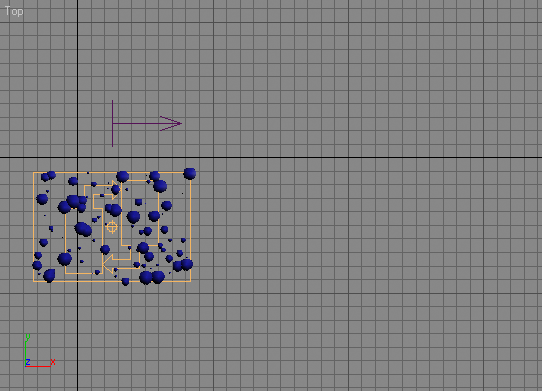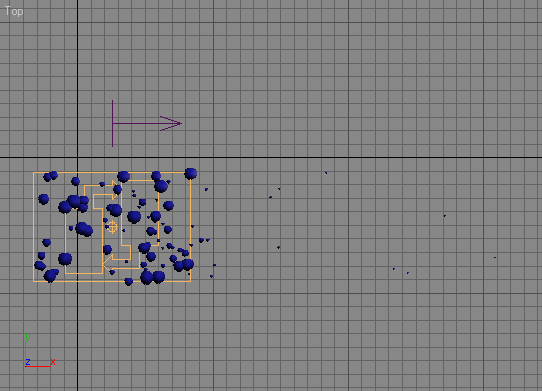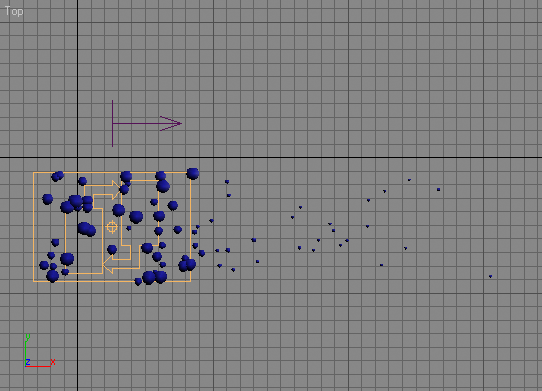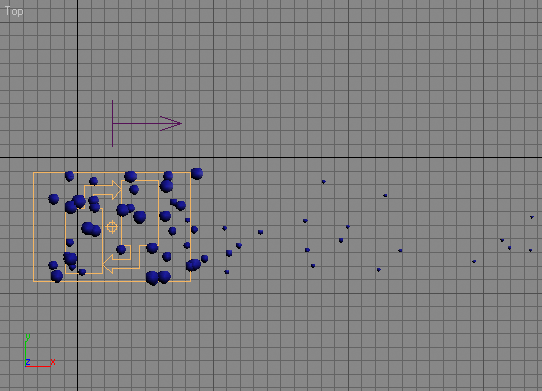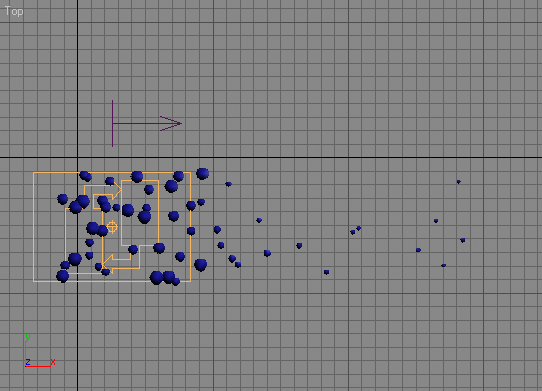Step-By-Step Comments

on ChannelsUsed pCont do
(

The ChannelsUsed handler defines the channels to be used by the Script Operator - you cannot get or set particle related values from the particle container without specifying which properties you need access to. This way, Particle Flow does not have to provide the Script Operator with all possible channels (and there can be an arbitrary number of channels in Particle Flow) but only with those that are actually needed. This conserves memory!
The parameter pCont contains  the Particle Container.

pCont.useScale = true

Since we want to read the size of the particles in order to calculate the mass, we will need access to the Scale channel.

pCont.useFloat = true

We will also need the Float channel which is a all-purpose data channel that can be used to store floating point values.

)

on Init pCont do
(

The Init  handler is used to initialize the Script Operator.
Usually you define variables and acquire initial values or create objects to be used by the Proceed handler.
In our case, we will not use this handler though.
The parameter pCont contains  the Particle Container.

)

on Proceed pCont do
(

The Proceed  handler is called every time the Script Operator is evaluated by Particle Flow.
It contains the actual body of the script.
The parameter pCont contains  the Particle Container which contains all particles the Operator is applied to.

count = pCont.NumParticles()

Here we read the current number of particles in the particle container.
We will access every one of them in the following loop.
The reason we assign the value to a variable is that in the for loop that follows, the to limit is evaluated after each cycle of the loop to decide whether the i variable is greater than the limit.
Using the pCont.NumParticles() method call inside the for loop would call the method n times where n is the number of particles.
With the current code though, the method will be called just once and this will make the script faster!

for i in 1 to count do
(

Now we repeat the following code block for every single particle by using a for loop which counts from 1 to the number of particles. The variable i will contain the current particle index.

pCont.particleIndex = i

In order to work with multiple particles, we have to specify the current particle to access.
Setting the .particleIndex property of the Particle Container to the i variable will make the i-th particle the current one.
Any subsequent particle property access calls will be directed to that particle!

pCont.particleFloat = 1.0/(pCont.particleScale^3)

This is the actual code line that calculates an influence value which is reverse-proportional to the mass of the particle.
The result is assigned to the particleFloat channel of the current particle and will be accessed by the Force Operator which has its Script Wiring enabled to accept the Float values from the particleFlot channel.

This line requires some more explanation.
In general, the mass of an object depends on its volume and its density.
Since we assume that all particles in this system are made of the same material, we don't really need the density.
We will use just the Bounding Sphere of the particles.
The volume of a sphere can be calculated using the following expression:

4/3*Pi*R^3

where R is the radius of the sphere.
Since 4, 3 and Pi are constants, we don't actually need them - they would just scale the final value a bit and make the calculation slower.
What we have now is Radius to the power of 3.
The influence should be decrease as the volume (and mass) increase, so we divide 1.0 by the R^3.

This is not completely realistic - when using Wind, the influence of the Wind is also proportional on the cross-section of the blown object.
The smaller the object, the lower the cross-section and thus lower the influence.
Since the cross-section of a sphere is a circle (Pi*R^2), we could reduce the R^3/R^2 to just R (which is the particleScale in our case)

So we should actually use the line

pCont.particleFloat = 1.0/pCont.particleScale

to be more realistic. Both functions provide a nice effect - feel free to use whatever suits your needs better.

)

This is the end of the i loop.

)

This is the end of the Proceed handler.

on Release pCont do
(

The Release handler is usually needed to do cleanup work, but we do not need it this time around.

)

 Copyright © 2003 by Borislav 'Bobo' Petrov.